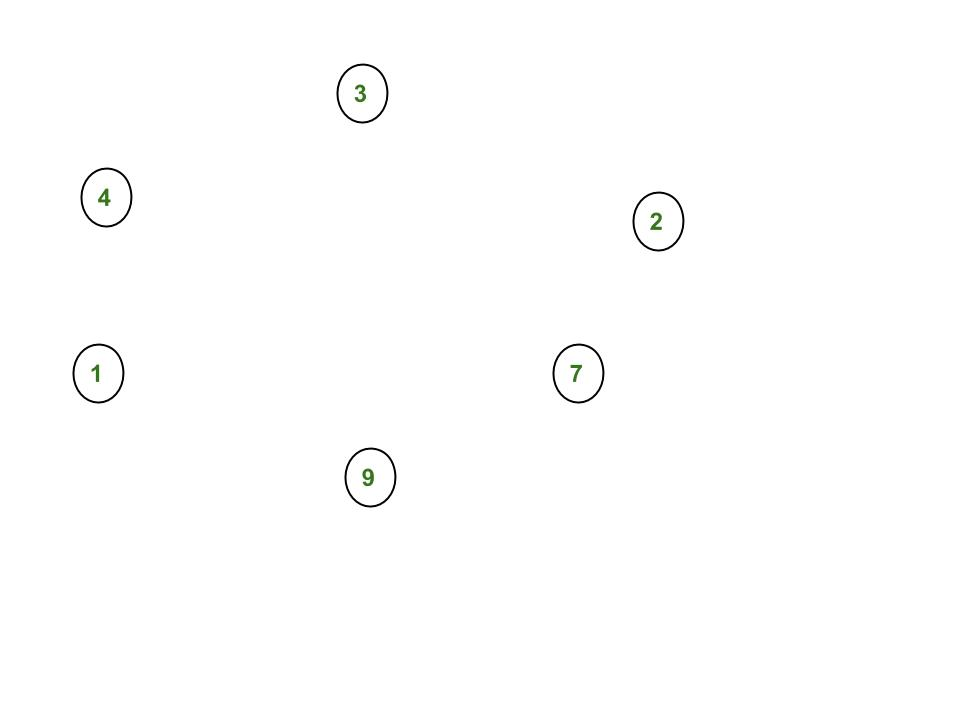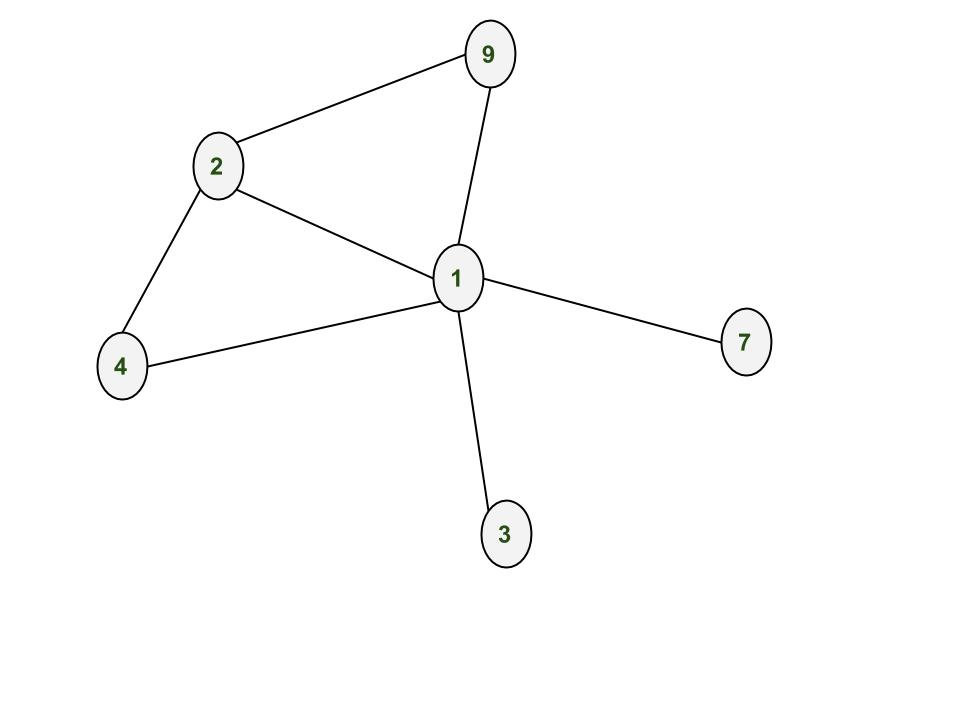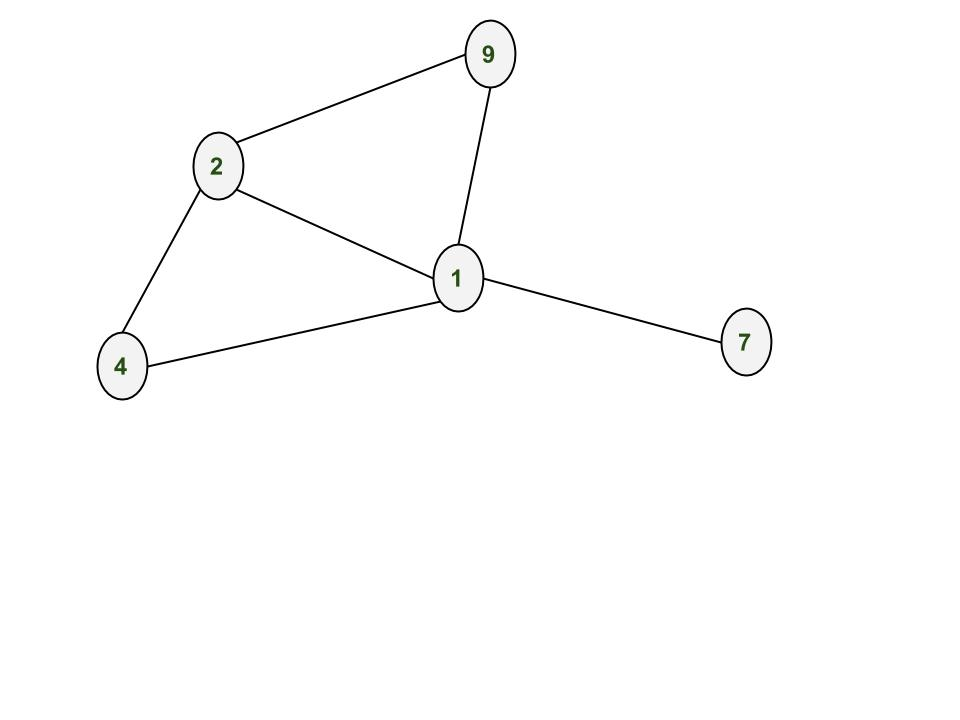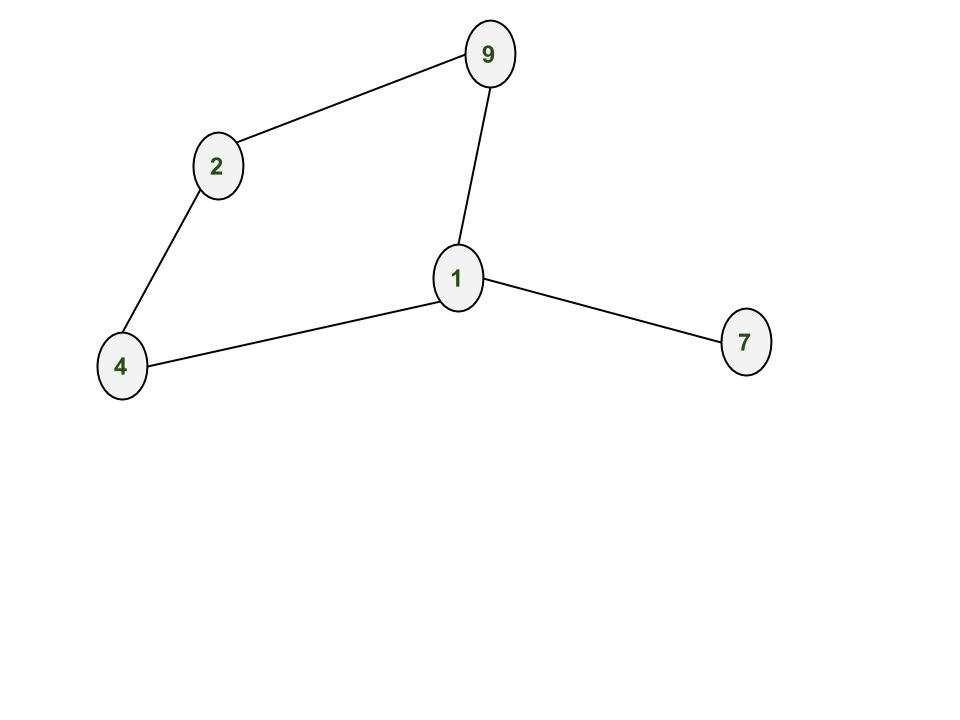# NetworkX : Python software package for study of complex networks

NetworkX is a Python language software package for the creation, manipulation, and study of the structure, dynamics, and function of complex networks. It is used to study large complex networks represented in form of graphs with nodes and edges. Using networkx we can load and store complex networks. We can generate many types of random and classic networks, analyze network structure, build network models, design new network algorithms and draw networks.
Installation of the package:

```pip install networkx
```

Creating Nodes

Add one node at a time:

` G.add_node(1)`

` G.add_nodes_from([2,3])`

Let us create nodes in the graph G. After adding nodes 1, 2, 3, 4, 7, 9Creating Edges

Adding one edge at a time:

```G.add_edge(1,2)

`G.add_edges_from([(1,2),(1,3)])`

After adding edges (1,2), (3,1), (2,4), (4,1), (9,1), (1,7), (2,9)Removing Nodes and Edges

One can demolish the graph using any of these functions:

```Graph.remove_node(), Graph.remove_nodes_from(),
Graph.remove_edge() and Graph.remove_edges_from()```

After removing node 3After removing edge (1,2)`# Python program to create an undirected  ` `# graph and add nodes and edges to a graph ` ` `  `# To import package ` `import` `networkx ` `  `  `# To create an empty undirected graph ` `G ``=` `networkx.Graph() ` `  `  `# To add a node ` `G.add_node(``1``) ` `G.add_node(``2``) ` `G.add_node(``3``) ` `G.add_node(``4``) ` `G.add_node(``7``) ` `G.add_node(``9``) ` `  `  `# To add an edge ` `# Note graph is undirected ` `# Hence order of nodes in edge doesn't matter ` `G.add_edge(``1``,``2``) ` `G.add_edge(``3``,``1``) ` `G.add_edge(``2``,``4``) ` `G.add_edge(``4``,``1``) ` `G.add_edge(``9``,``1``) ` `G.add_edge(``1``,``7``) ` `G.add_edge(``2``,``9``) ` `  `  `# To get all the nodes of a graph ` `node_list ``=` `G.nodes() ` `print``(``"#1"``) ` `print``(node_list) ` `  `  `# To get all the edges of a graph ` `edge_list ``=` `G.edges() ` `print``(``"#2"``) ` `print``(edge_list) ` `  `  `# To remove a node of a graph ` `G.remove_node(``3``) ` `node_list ``=` `G.nodes() ` `print``(``"#3"``) ` `print``(node_list) ` `  `  `# To remove an edge of a graph ` `G.remove_edge(``1``,``2``) ` `edge_list ``=` `G.edges() ` `print``(``"#4"``) ` `print``(edge_list) ` `  `  `# To find number of nodes ` `n ``=` `G.number_of_nodes() ` `print``(``"#5"``) ` `print``(n) ` `  `  `# To find number of edges ` `m ``=` `G.number_of_edges() ` `print``(``"#6"``) ` `print``(m) ` `  `  `# To find degree of a node ` `# d will store degree of node 2 ` `d ``=` `G.degree(``2``) ` `print``(``"#7"``) ` `print``(d) ` ` `  `# To find all the neighbor of a node ` `neighbor_list ``=` `G.neighbors(``2``) ` `print``(``"#8"``) ` `print``(neighbor_list) ` ` `  `#To delete all the nodes and edges ` `G.clear()`

Output :

```#1
[1, 2, 3, 4, 7, 9]
#2
[(1, 9), (1, 2), (1, 3), (1, 4), (1, 7), (2, 4), (2, 9)]
#3
[1, 2, 4, 7, 9]
#4
[(1, 9), (1, 4), (1, 7), (2, 4), (2, 9)]
#5
5
#6
5
#7
2
#8
[4, 9]
```

In the next post, we’ll be discussing how to create weighted graphs, directed graphs, multi graphs. How to draw graphs. In later posts we’ll see how to use inbuilt functions like Depth fist search aka dfs, breadth first search aka BFS, dijkstra’s shortest path algorithm.

Reference :
Networxx at Github

This article is contributed by Pratik Chhajer. If you like GeeksforGeeks and would like to contribute, you can also write an article using contribute.geeksforgeeks.org or mail your article to contribute@geeksforgeeks.org. See your article appearing on the GeeksforGeeks main page and help other Geeks.

GeeksforGeeks has prepared a complete interview preparation course with premium videos, theory, practice problems, TA support and many more features. Please refer Placement 100 for details

My Personal Notes arrow_drop_up
Article Tags :
Practice Tags :

1

Please write to us at contribute@geeksforgeeks.org to report any issue with the above content.